# 15.10 - Discovering Hash and Join Indexes for Derived Statistics - Teradata Database

## Teradata Database SQL Request and Transaction Processing

prodname
vrm_release
15.10
category
Programming Reference
User Guide
featnum
B035-1142-151K

To support the use of join indexes for derived statistics, the Optimizer locates all single-table sparse join indexes whose cardinality and statistics are potentially useful for estimating values such as the selectivity of base table conditions and column correlations. Teradata Database also gathers together any non‑sparse single‑table join indexes and hash indexes that can be used for inheriting statistics by the base table.

For a given query, the Optimizer identifies all single‑table join indexes that can cover a query predicate. Also see “Using Join Index Statistics to Estimate Single‑Table Expression Cardinalities” on page 260 for information about how the Optimizer can use single‑table join index statistics to estimate the cardinalities of complex expressions written on base table columns.

The select list and GROUP BY clause of the query are ignored at the discovery phase, and early coverage analysis is based only on its predicates. The Optimizer captures and retains the column mapping information from join indexes to the base table field IDs so they are available for qualifying the join index for different derived statistics applications.

The Optimizer searches for join indexes whose statistics or cardinality or both are usable for single-table condition selectivity estimates once per flattened query block in the prejoin planning stage. The qualified join indexes are then stored and can later be used for the planning of different join index rewrites.

For example, assume a query references tables t1, t2, t3, t4 with single-table join indexes j1 and j3 defined on t1 and t3, respectively.

The Join Planner can use these join indexes in the following three ways.

• The original query {t1, t2, t3, t4} can use the statistics from both j1 and j3.
• The rewritten query {j1, t2, t3, t4} can use the statistics from j3.
• The rewritten query {t1, t2, j3, t4} can use the statistics from j1.
• The following cases describe how the non‑aggregate single‑table sparse join indexes are used to help make single-table cardinality estimates. Also see “Using Join Index Statistics to Estimate Single‑Table Expression Cardinalities” on page 260 for information about a different form of cardinality estimation using hash or join index statistics.

• The join index predicates are a subset or identical to those of the query predicates. For example, suppose you have the following query and join index definitions.
• `     SELECT * `
`     FROM t1 `
`     WHERE a=10 `
`     AND   b BETWEEN 20 AND 30;`
` `
`     CREATE JOIN INDEX ji AS`
`       SELECT a `
`       FROM t1 `
`       WHERE b BETWEEN 20 AND 30;`

Even without an interval histogram on ji1, the Optimizer can use the cardinality of the join index as the number of rows selected by the predicate` b BETWEEN 20 AND 30`.

The selectivity of the predicate `b BETWEEN 20 AND 30` can be calculated by dividing the join index cardinality by the base table cardinality. However, without the base relation interval histograms, the qualified number of values and the high modal frequency of the column set must be derived from other sources.

The Optimizer can use a histogram on columns projected from the join index to estimate the selectivity of the residual conditions of a query that are not covered by the conditions of that join index. The combined selectivity of the matching and residual conditions that use join index statistics is the selected rows cardinality determined by applying residual terms divided by the base table cardinality.

For the preceding example of ji1, the Optimizer can use the histogram on ji1.a to find the number of rows that qualify `a=10`, which is also the number of rows that qualify for the combined predicate `a=1 AND b BETWEEN 20 AND 30`.

Therefore, the combined selectivity is calculated as follows.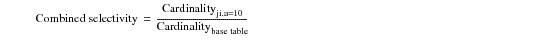where:

 Equation element … Specifies the number of rows … Cardinalityji.a=10 from ji that satisfy the predicate `ji.a=10`. Cardinalitybasetable in the base table.

See “Using Join Index Statistics to Estimate Single‑Table Expression Cardinalities” on page 260 for information about how the Optimizer can estimate the cardinalities of single‑table complex expressions using single‑table join index statistics.

• The join index contains all rows required to answer the query, but its predicates do not match the query predicates. In this case, the join index is usable for cardinality estimation purposes only if it has interval histograms for all the columns referenced in the non‑matching conditions of the query. For example, suppose you have the following query and join index definitions.
• `     SELECT * `
`     FROM t1 `
`     WHERE a=10 `
`     AND   b BETWEEN 20 AND 30 `
`     AND   c=11;`
` `
`     CREATE JOIN INDEX ji2 AS`
`       SELECT a `
`       FROM t1 `
`       WHERE a>5 `
`       AND   b BETWEEN 20 AND 30;`

The Optimizer uses ji2 for selectivity estimation only when there is an interval histogram on ji2.a, and the combined selectivity of `a=10 AND b BETWEEN 20 AND 30` must be calculated using ji2.

The combined selectivity of `a=10 AND b BETWEEN 20 AND 30` is, as before, calculated using the following equation: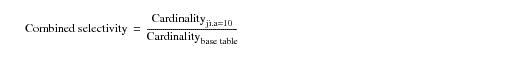The selectivity calculated using the cardinality or interval histogram of one join index is combined with selectivity calculated from other sources such as base table interval histograms or another join index.

In this case, although ji2 cannot be used to calculate the selectivity of `a=10 AND b BETWEEN 20 AND 30`, the cardinality of ji2 can be used as the upper bound on the rows selected by the predicates `a=10 AND b BETWEEN 20 AND 30` or the entire WHERE clause for the query.

The Optimizer uses the number of unique values and the high modal frequency of join columns to make join cardinality, rows per value, skew, and other estimates. For example, consider the following query:

`     SELECT * `
`     FROM t1, t2`
`     WHERE x1= x2 `
`     AND   y1=10;`

The number of unique values on x1 after the predicate `y1=10` is applied is crucial data for more accurately estimating the join cardinality, rows per value, and so on. The number of unique values of x1 after applying the predicate `y1=10` is called the correlated values of x1 given y1=10.

A base table histogram on x1 can only provide the estimate before condition `y1=10` is applied. Even a multidimensional histogram on (x1, y1) might not always provide accurate correlation information because of information missing from the multicolumn statistics such as column ordering, length limits on concatenated column values, and so on.

Single-table join indexes can be used to calculate qualified demographic information for join columns or grouping columns after applying the single‑table conditions. In the previous example, if there is a join index ji3, defined as follows, it is possible to use the interval histogram for ji3.x1 to make an accurate estimate of the number of unique values and high modal frequency on x1.

`     CREATE JOIN INDEX ji3 AS    `
`       SELECT x1 `
`       FROM t1 `
`       WHERE y1=10;`

Using the interval histogram for ji3.x1, the Optimizer can make accurate estimates of the number of unique values and high modal frequency of x1. This information is collected and propagated by the derived statistics framework.

Teradata Database can use a join index for deriving the column correlations when either of the following conditions is satisfied:

• The join index predicates are a subset of the query predicates.
• The join index predicates are an exact match to the query predicates.
• When the join index has the same set of predicates as the single-table predicates in the query, the column correlation estimates are very accurate. In this case, the join index contains more rows than necessary to answer the query (satisfying the first bullet in the list), the estimate based on the join index histogram is still better than using the base table interval histogram statistics because the base table histogram represents the entire domain, whereas the join index histogram represents the domain only after applying single-table conditions.

The number of unique values plays an important role for estimating the join cardinality, rows per value, or number of rows returned by an aggregation operation or query. For example, suppose you have the following query:

`     SELECT x, y, SUM(z)`
`     FROM t1`
`     WHERE a=10 `
`     AND   c>20`
`     GROUP BY x, y;`

The cardinality of this query equals the number of unique values of (x, y) after applying the WHERE clause predicate `a = 10 AND c > 20`. Two kinds of join indexes are useful for estimating cardinalities for this query.

• A join index with the same single-table predicate and GROUP BY columns as the join index ji4.
• The cardinality of ji4 is equal to the cardinality of the preceding query.

`     CREATE JOIN INDEX ji4 AS`
`       SELECT x, y, COUNT(z)`
`       FROM t1`
`       WHERE a=10 `
`       AND c>20`
`       GROUP BY x, y;`
• A join index with the same single-table predicate as the join index and specifying any GROUP BY clause.
• The cardinality of the join index, which can be determined either by dynamic AMP sampling or from its primary index statistics, is the number of unique values of the grouping columns after applying the single-table conditions. The derived unique values can then be used in the join planning of the query. For example, the number of unique values of (x1, y1) derived from the cardinality of ji5 can be used in the join cardinality estimation of the query.

Consider the following query and join index definitions:

`     SELECT * `
`     FROM t1, t2`
`     WHERE x1=x2`
`     AND   y1=y2 `
`     AND   a=10`
`     AND   c>20;`
`     `
`     CREATE JOIN INDEX ji5 AS`
`       SELECT x1, y1 `
`       FROM t1 `
`       WHERE a=10 `
`       AND   c>20 `
`       GROUP BY 1, 2;`

The Optimizer allocates an entry for the grouping columns of a query in the derived statistics framework if one does not already exist. Its number of unique values is the cardinality of the applicable aggregate join index, and that value is propagated forward so it can be used later in join planning.

When the aggregate join index predicates are a subset of the query predicates, the Optimizer can still use the estimate of the number of unique values derived from the aggregate join index because it is more reasonable than the estimate from the base table interval histogram.

Single-table cardinality estimation is independent of the Access Path Planner. Such cardinality estimates can be derived from any of the following bases:

• Single-table join indexes for cardinality estimates.
• Derived statistics, which has the information from domain‑based constraints such as CHECK and referential integrity constraints.
• Complex single-table predicates.
• Combined multiple selectivities can handle overlapping and selectivities derived from different sources such as join index interval histograms and derived statistics.
• Whenever possible, column correlations collected from single-column and multicolumn statistics are used in combining selectivities.
• Single‑table cardinality estimation uses single-table join indexes, particularly when estimating the single‑table cardinalities of complex expressions (see “Using Join Index Statistics to Estimate Single‑Table Expression Cardinalities” on page 260).

An expression that can use histograms for cardinality estimation can contain either a unary or a binary operator. One of the operands must be either a column or a built-in functions or SQL operator from the following lists:

• UPPER
• LOWER
• NULLIFZERO
• ZEROIFNULL
• SUBSTR
• MOD
• CONCAT on columns of same table
• Implicit or explicit data type conversions on a column
• The supported operators are the following:

• =
• >=
• <=
• >
• <
• <>
• IS NULL
• IS NOT NULL
• LIKE
• NOT LIKE
• BETWEEN
• IN
• For binary operators, the other operand can be a simple expression involving any of the following:

• Constants whose value can be calculated by the Parser.
• System USING request modifier data or built‑in function data such as a CURRENT_DATE value.
• Simple expressions involving another column from the same table.
• For example, the selectivity of the single‑table predicate` t1.x1 = t1.y1` can be estimated more reasonably by considering the overlapping values of those 2 columns than by using a default selectivity formula.

Selectivity estimates for LIKE predicates is limited to "abc%" patterns and very conservative estimation formulas.

The default ratio for “abc%” patterns can be adjusted in your Cost Profile by setting the value for the LIKEEstPartialIntRatio constant to enable LIKE predicate selectivity to be derived from a partially covering interval in an interval histogram (see “Optimizer Cost Profiles” on page 307). LIKEEstPartialIntRatio specifies the fraction of the applicable rows that the system assumes satisfies the constraint. The applicable rows can be the entire table for a predicate like `WHERE descrip LIKE '%abc%'`, or they can be a subset of the table for a predicate like `WHERE lastname LIKE 'abc%'`. In the case of a proper subset, and having collected histogram statistics, the Optimizer considers only the applicable intervals. The default value is 0.125 of the qualified rows. This default lowers the predicted number of rows that match a LIKE condition from the
Type 1 default, and biases join plans toward a duplicated join geography (see “Join Distribution Strategies” on page 365). If you experience a performance regression because the system underestimates the number of rows matching a LIKE condition using the default LIKEEstPartialIntRatio value of 0.125, which then causes a different spool table redistribution to be chosen by the Optimizer, you can create a variable cost profile with a larger LIKEEstPartialIntRatio value to compensate.

There can be no single optimal value for this flag. The best value for LIKEEstPartialIntRatio depends on the table and the constant in a particular SQL request. You would anticipate, for example, that constraints such as `WHERE sex LIKE 'fe%'` and `WHERE lastname LIKE 'jingleheimershmit%'` would differ greatly in their respective selectivities, and it is not possible for one value of LIKEEstPartialIntRatio to be the single best value for all SQL requests across all system workloads.

The following table indicates one possible way to handle the issue of widely varied predicate selectivities for the typical workloads submitted by multiple users:

The Optimizer considers full and partial coverage of all covering intervals individually, making it possible to make the selectivity calculations for “abc%” patterns still more accurate.

The Optimizer can use a multicolumn histogram for a group of predicates when the following statements are all true:

• The predicates are specified on the first n fields of the multicolumn histogram.
• This rule exists because there is an ordering dependency of the fields of a multicolumn histogram.

• The predicates must specify an equality condition except for the first field of the multicolumn histogram.
• If the predicate on the first column of a multicolumn is a non‑equality condition, then the Optimizer uses the multicolumn histogram for this predicate only.
• For example, a histogram on (x, y, z) can be used to estimate the selectivity for predicate `x>100` as well as `x=10 AND y=20`.

The Optimizer can also use the data derived by date extrapolation to enhance its cardinality estimates for date‑related predicates (see “Using Extrapolation to Replace Stale Statistics” on page 288).

The Optimizer can detect independent columns and calculate their combined selectivity as the product of their individual selectivities. Two categories of independent columns are defined.

• Every value of one column maps to all values of the other column.
• In this case, the number of combined values is the product of the number of values of individual column. Take column nation and column market-segment of the customer table as an example.

Each nation participates in the business of all market segments, while business in each market segment is provided in all countries.

During cardinality estimation, 2 columns are considered to be independent if their number of combined values is close to the number of values of the individual column. That is, column c1 and c2 are independent if their number of unique values satisfies the following inequality: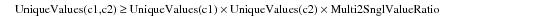where:

 Equation element … Specifies … UniqueValues (c1,c2) Combined number of unique values in the column set (c1,c2). UniqueValues (c1) Number of unique values in column c1. UniqueValues (c2) Number of unique values in column c2. Multi2SnglValueRatio The value of the Multi2SnglValueRatio flag in your cost profile. By default, this value is 0.9.
• The value of one column is not constrained by the value of the other column, but given a value of the first column of 2 independent columns, the possible value of the first column is evenly distributed across all values of the second column.
• Column market-segment and column account-balance of the customer table are an example of such independent columns. On the one hand, the account balance is not bounded by a specific market segment even though one account balance, say \$100.23, does not exist in all market segments.

The following example is a sample of the histogram for (account‑balance, market‑segment):

` Interval`
`   Max. Value: -341.39,'AUTOMOBI'`
`   Mode Value: -349.49,'MACHINER'`
` Interval`
`   Max. Value: -281.02,'FURNITUR'`
`   Mode Value: -312.03,'MACHINER'`
` Interval`
`   Max. Value: -226.32,'BUILDING'`
`   Mode Value: -259.31,'BUILDING'`
` `
`…`
` `
` Interval`
`   Max. Value: 9082.16,'MACHINER'`
`   Mode Value: 9057.08,'AUTOMOBI'`
` Interval`
`   Max. Value: 9134.95,'FURNITUR'`
`   Mode Value: 9089.16,'AUTOMOBI'`
` Interval`
`   Max. Value: 9189.65,'FURNITUR'`
`   Mode Value: 9153.14,'HOUSEHOL'`

For this kind of value independency, the Optimizer uses double safety tests to detect value independence. For the 2 algorithm described in the following paragraphs, assume that single‑column statistics have been collected on (c1), (c2) and multicolumn statistics have been collected on (c1,c2).

• Algorithm1
• If 2 columns are independent, given any 2 widely separated values of c1, the value ranges of a corresponding column c2 still overlap with the 2 values of c1. This can be done by sampling 2 blocks of equal-height intervals in multicolumn statistics.

Assume the first block is from interval k to k+n, and the second block is from interval j to j+n. Make sure that the 2 blocks have some gaps such that j > k+n+p. Check whether the ranges of c2 in those 2 blocks of intervals overlap.

For instance, in the histogram of (account-balance, market-segment), the value range of market-segment in Interval 12 to Interval 14 is from 'AUTOMOBI' to 'MACHINER', while that of Interval 183 to Interval 185 is also 'AUTOMOBI' to 'MACHINER'.

The value ranges overlap, so the algorithm is valid.

• Algorithm 2
• If 2 columns are independent, then given any value of c1, the range of c2 should be closed to the whole range of c2.

This can be done by finding the max/min value of column c2 from interval k to interval k+n of multicolumn statistics (c1,c2), and then calculating the selectivity of `c2 BETWEEN min AND max` using the histogram for c2.

The 2 columns are independent if the selectivity of `c2 BETWEEN min AND max` is greater than CCFIndependentValueRatio, which by default is 0.6.

For instance, in the histogram of (account-balance, market-segment), the minimum value of market-segment in both interval blocks is 'AUTOMOBI' and the maximum value is 'MACHINER'. The selectivity of `market-segment BETWEEN 'AUTOMOBI' AND 'MACHINER'` is 1.0 from the histogram on the market-segment column.

To be conservative, the system assumes that 2 columns are independent only if the outcomes of both Algorithm 1 and Algorithm 2 determine that the 2 columns are independent. The algorithm can also be used for 2‑column sets, not just 2 single columns.

There are three cases where the Optimizer cannot detect column independence.

• When the total size of all columns exceeds the histogram data row size limit of 16 bytes. In this case, multicolumn histogram does not have all the information necessary to make an accurate determination of column independence, so the test for independence could fail.
• When independence detection is activated only when the confidence of the individual selectivities is high. That is, for predicates whose selectivity is not high confidence because no statistics have been collected on the columns, or because an expression is complex, the Optimizer does not attempt to detect column independence.
• When independence detection is activated only when the selectivity estimation of a predicate on an individual column is based on the base table histogram.
• To more easily study this case, assume that both column X and column Y are evenly redistributed.

1 Given that Y value d1 maps to X value (c1, c2…, cm), it is possible to calculate the number of rows selected by the predicate (y=d1 AND x=c1) similar to the second case in the previous topic.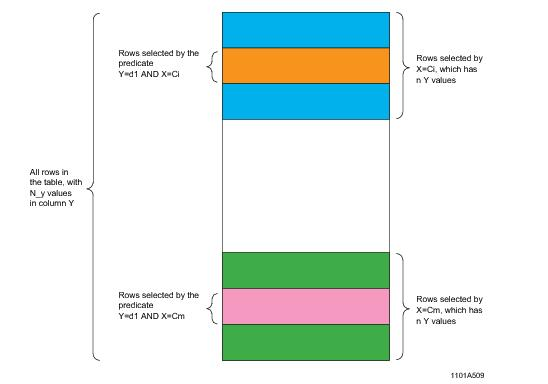2 Because Y is evenly distributed, each Y value maps to same number of rows, which is the RowsPerValue, or RPV.

From the perspective of the whole table,From the perspective of the rows selected by the predicate (x=ci),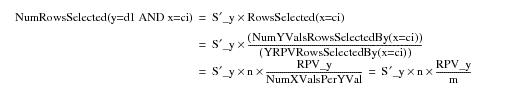3 Because the same set of rows is selected in both cases,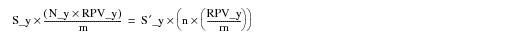Rearranging and eliminating terms,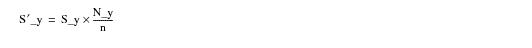and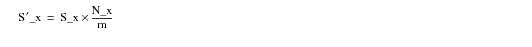4 This means that the combined selectivity is calculated in either of the following ways.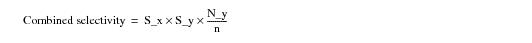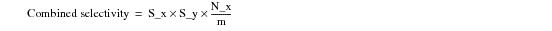5 When both X and Y are evenly distributed and the value mapping from X to Y is m:n, it follows that and therefore .

To avoid the anomaly, the combined selectivity has an upper bound equal to the more highly selective of the two.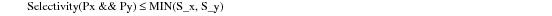This is because a conjoined predicate should never increase the selectivity of the other term.

The following table summarizes these results.

 Predicate Px and Py From Same Column? Selectivity(Px AND Py) Comments Yes 0 Except range constraints No S_x * S_y * N_y/n or S_x * S_y * N_x/m When value mapping from X to Y is m:n. If Selectivity(Px AND Py) > MIN(S_x, S_y), then set it to MIN(S_x, S_y) No AndFF(MIN(S_x, S_y), MAX (F, S_x, S_y)) A stochastic model based selectivity combination algorithm in AndFF() is used when no column correlation is defined or discovered between X and Y, where F is the fudge factor.

The combined selectivity of two ORed predicates can be derived from their corresponding ANDed predicates.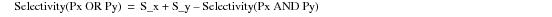A predicate is redundant if the evaluation of other predicates determines an identical result. If redundant predicates are not detected and handled properly during join planning, they can adversely affect cardinality and costing estimates, leading to non‑optimal query plans. Redundancy can occur either from internally‑generated predicates derived from transitive closure (see “Predicate Simplification” on page 94) or from user‑specified SQL predicates.

Transitive closure generates new predicates if the set of predicates given in the query logically implies other predicates. The added predicates provide more opportunities for single-table access paths and join planning.

For example, given the following query.

`     SELECT * `
`     FROM t1, t2, t3 `
`     WHERE t1.x1 = t2.x2`
`     AND   t2.x2 = t3.x3;`

Through transitive closure, the system can generate a third predicate, as follows.

`     SELECT * `
`     FROM t1, t2, t3 `
`     WHERE t1.x1 = t2.x2`
`     AND   t2.x2 = t3.x3`
`     AND   t1.x1 = t3.x3;  ← Derived predicate`

Assume the join plan is as follows.

`     j1 (t1 X t2) ON (x1 = x2)`
`     j2 (j1 X t3) ON (x2 = x3 AND x1 = x3)`

where X indicates the JOIN operator.

When joining (`j1 `X` t3`), one of the pair of join conditions is redundant, because evaluating either individually produces the same result set. This is because the previous join had already equated x1 to x2 using the condition (`x1=x2`).

The Optimizer algorithms that use the number of unique values on join columns recognize this, and consider the number of unique values for column combination (j1.x1, j1.x2) as the minimum number of values of x1 and x2 based on the join uniformity assumption. Redundancy detection logic recognizes this, so it ignores the condition that has the greater number of unique values for unique value estimates.

In this example, if t1.x1 has more unique values, then the Optimizer ignores the last condition, (`x1=x3`).

Redundancy detection works with derived statistics as follows.

After each join, whether temporary or committed, the Optimizer takes the following actions.

1 The connected components, or equated columns, using join predicates are grouped together and marked as EquiSets (see “Using Join Predicate Redundancy to Derive Column Demographics After Each Binary Join” on page 236 for a definition of EquiSets). These groups are either added as EquiSet entries to the derived statistics or merged with other EquiSets if they intersect. The individual column entries that formed the EquiSet are then removed from further consideration in the join plan.

The demographic information such as number of unique values and High Modal Frequency is derived as follows and saved as part of derived statistics entry.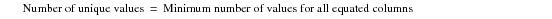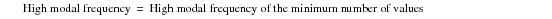Demographic information from EquiSet entries can be used either for an exact match or for a match on any subset of its columns. The next stage in the process is made aware of the EquiSet entries to enable it to make use of them in the most efficient way it can. The EquiSets also help aggregation estimates and outer block join planning because the system propagates them to outer blocks of the query.

For example, after join j1 in the previous example, the EquiSet is (j1.x1, j2.x2). This set can then be used to derive column demographics for j1.x1, j2.x2, and the combination (j1.x1, j2.x2).

2 The Join Planner might need to know the demographics of the different combinations of columns. To help the Optimizer with subsequent join planning, many combinations of entries can be derived if an EquiSet intersects with other multicolumn statistics entries.

For example, if there is a multicolumn statistics entry (j1.x1, j1.y1) and an EquiSet (j1.x1, j1.x2), the following entries are the 2 equivalent entries that can use the demographic information from the multicolumn statistics.

`   `(j1.x1, j1.x2, j1.y1)  By augmenting with the connected column

`   `(j1.x2, j1.y1)          ←  By replacing the connected column

If many multicolumn entries intersect with many EquiSets, there can be an exponential growth in the number of derived statistics entries because of the additional equivalent combinations.

On the one hand, the large number of entries made during redundancy analysis makes it easier to select the best combinations in a single scan.

On the other hand, the possibly exponential growth of entries can cause excessive memory consumption and longer compilation times, so the responsibility of identifying equivalent entries is given to whichever optimization stage that uses the derived statistics at each stage of the process.

3 To be able to derive EquiSets, the system needs to have single column statistics on at least one operand of the join predicate.

4 If the only available statistics are multicolumn statistics that cover the join columns for both of the input relations, the system makes a regular derived statistics entry with all the columns, using the demographics of the minimum values multicolumn statistics.

For example, given the predicate `x1=x2 AND y1=y2`, and multicolumn statistics on
(x1, y1) and (x2, y2), the system makes the derived statistics entry (x1, y1, x2, y2) after the join, with the minimum number of values of [(x1, y1), (x2, y2)]. Note that this is not an EquiSet because it cannot be used for any subset of its columns.

5 The derived entries are propagated to the subsequent joins and stages as required, based on the required columns from column projections. The entries can also be expanded based on subsequent join conditions during the remainder of join planning.

Note that selectivity redundancy is detected only for inner joins with equality join predicates.

Consider the following example.

`     SELECT * `
`     FROM t0,(SELECT t1.x1, t2.x2, t2.x3, t2.y2, MAX (t2.z2) `
`              FROM t1, t2, t3`
`              WHERE t1.x1=t2.x2`
`              AND   t2.x2 = t3.x3`
`              AND   t1.x1 = t3.x3   ← derived condition`
`              AND   t2.y2 = t3.y3 `
`              GROUP BY 1, 2, 3,4) DT(x1, x2, x3, y2, mx)`
`     WHERE t0.x0 = dt.x1 `
`     AND   t1.y0 = dt.x2;`

The processing of this query is shown in the following graphic.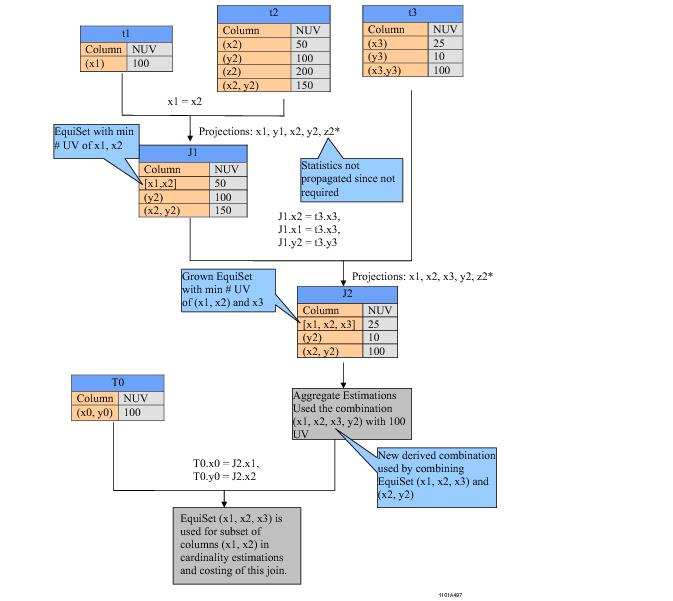where:

 Graphic element … Specifies … NUV number of unique values.

Join cardinality estimates, rows per value estimates, skew detection, aggregate estimates, Partial Group By estimates, and several other computations all require estimating the number of unique values for a given set of join or grouping columns.

The Optimizer makes an exhaustive search of all possible combinations of statistics to determine the best set of non‑overlapping statistics. Once it has been decided, that set is then used to find the Number of Unique Values, High Modal Frequency, and High AMP Frequency values at all stages of the optimization process.

The major goals of this algorithm are as follows.

• Find the set of non‑overlapping combinations of statistics that has the least number of unique values.
• If no complete coverage can be found, find the set of non‑overlapping combinations of statistics that covers the largest number of columns and has the least number of unique values.

• Find the set of non‑overlapping combinations of statistics that provides the highest High Modal Frequency and High AMP Frequency values by covering all the columns.
• The set of combinations that provides the smallest Number of Unique Values might not be the same set that provides the best estimates for the High Modal Frequency and High AMP Frequency values because the High Modal Frequency and High AMP Frequency might not be available for some of the entries that are derived from sources other than base table interval histograms.

The number of unique values determined from partial statistics, where statistics do not cover all the hash or join columns, is considered for Rows Per Value and join cardinality estimates because it is a conservative approach, but the Optimizer does not consider this estimate for skew adjustment and Partial GROUP BY because it makes very aggressive cost estimates and, as a result, can cause performance problems when the costing estimates are grossly in error because of the overly aggressive method by which they were calculated. In other words, the Optimizer avoids Partial GROUP BY plans and does not attempt to make skew adjustments when the given hash or join columns are not fully covered by statistics.

The unique values discovery algorithm provides the following information (see “EXPLAIN Confidence Levels” on page 537 for the definitions of the various Optimizer confidence levels for making cardinality estimates).

• MinVals and its confidence level
• This estimate provides the absolute minimum number of values for the given collection of columns. The values are taken from a single derived statistics entry that covers the largest number of columns. If there are multiple entries that cover the same number of columns, the Optimizer selects the entry with the highest number of values.

The confidence levels for various entries are described in the following table.

 IF a usable derived statistics entry is … The confidence level is … found High confidence not found No confidence
• BestVals and its confidence level
• This provides the best number of values estimate that can be derived from the set of derived statistics entries that meets both of the following criteria.

• Covers the greatest number of columns
• Produces the least number of values
• Derived statistics entries that are either the same set, or a subset, of the given column collection are used to produce these values.

The values can even be taken from a set of derived statistics entries that covers only a portion of the columns in some cases. This can happen when, for example, there are insufficient statistics to cover all the columns.

The confidence levels for various entries are described in the following table.

 FOR this derived statistics entry situation … The confidence level is … a single entry covers all the columns High confidence either of the following. multiple entries must be combined to cover all the columns only a subset of the columns is covered Low confidence no usable derived statistics entries are found No confidence
• MaxVals and its confidence level
• This estimate provides the maximum number of possible values for the given collection of columns. The derived statistics entries that are either a subset, a superset, an EquiSet, or intersecting entries are all considered for producing these values.

If all columns are not covered by these entries, then default estimates based on the domain type are used for the non‑covered columns, as described in the following table.

 FOR this derived statistics entry situation … The confidence level is … a single entry covers all the columns High confidence multiple entries must be combined to cover all the columns Low confidence default estimates are used to compute the values No confidence

If MinVals is found with a High or Low level of confidence, then the Optimizer can always determine a BestVals statistic. However, it is also possible to determine MaxVals with High or Low confidence, but not be able to determine a BestVals or MinVals.

The Optimizer uses BestVals for its join cardinality and RowsPerValue estimates if it has Low or High confidence.

Partial GROUP BY, aggregations, and skew detection always use MaxVals.

The values are combined in 2 levels using a stochastic model.

1 The values from the same source are combined.

The number of combined values is limited to the total rows of the source.

2 The combined values from different sources are themselves combined to get the final values.

These are limited based the total rows for the current set.

The system consumes the EquiSet entries and then derives additional combinations if any multicolumn statistics intersect with the EquiSets. For example, if the usable entries for the given hash columns (x1, x2, y2) are EquiSet [x1, x2] and (x2, y2)], then the Optimizer augments the multicolumn statistics entry (x2, y2) with other EquiSet columns, producing the new entry (x1, x2, y2) dynamically.

The following examples describe the different possibilities.

For the first example, consider the following derived statistics entries.

 Column Set Number of Unique Values (a1, b1) 10 (b1, c1) 15 (a1, b1, c1) 20

If the hash columns are (a1, b1, c1), then the Optimizer derives the following values and confidence levels.

 Statistic Value Confidence Level MinVals 20 High confidence BestVals 20 High confidence MaxVals 20 High confidence

For the second example, consider the following derived statistics entries.

 Column Set Number of Unique Values (a1, b1) 10 (c1) 5

If the hash columns are (a1, b1, c1, d1), then the Optimizer derives the following values and confidence levels.

 Statistic Value Confidence Level MinVals 10 High confidence BestVals 50 Calculated from the product of the numbers of unique values for the column sets: 10 x 5 = 50. Low confidence MaxVals Combination of 50 and the demographic estimate for d1. No confidence

For the last example, consider the following derived statistics entry.

 Column Set Number of Unique Values (a1, b1, c1, d1) 100

If the hash columns are (a1, b1, c1), then the Optimizer derives the following values and confidence levels.

 Statistic Value Confidence Level MinVals TotalRows No confidence BestVals TotalRows No confidence MaxVals 100 Low confidence

The following flow chart shows the framework used to determine all these estimates and their accompanying costing logic.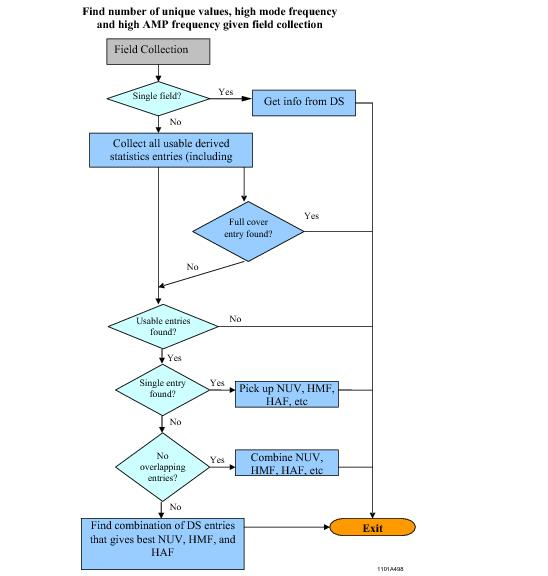where:

 Graphic element … Specifies … DS Derived Statistics NUV Number of Unique Values HMF High Modal Frequency HAF High AMP Frequency